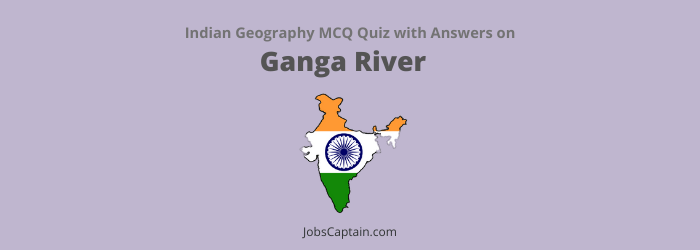# MCQ Quiz on Ganga RiverLet’s take an online MCQ test on Ganga River System or Ganges Drainage System.

Question 1. River Ganga, after entering Bangladesh is known by which of the following names?

(A) Nabaganga

(B) Kaliganga

(D) Lohit

Question 2. Sunderban Delta is formed by the rivers _________.

(A) Ganga and Sindhu

(B) Sindhu and Jhelam

(C) Ganga and Jhelam

(D) Ganga and Brahmaputra

(D) Ganga and Brahmaputra

Question 3. The Ganga river is example of ___________.

(A) Subsequent Drainage System

(B) Superimposed Drainage System

(C) Consequent Drainage System

(D) The Antecedent Drainage

(D) The Antecedent Drainage

Question 4. In Bangladesh River Ganges is known as __________.

(A) Mahaganga

(B) Bhagirathi

(D) Meghana

Question 5. The depth of Gangetic alluvial soil below the land surface is about …

(A) 800 metre

(B) 800 metre

(C) 600 metre

(D) 6000 metre

(D) 6000 metre

Question 6. Which of the following is the place of confluence of the Alaknanda and the Bhagirathi?

(A) Dev Prayag

(B) Rudra Prayag

(C) Karna Prayag

(D) Vishnu Prayag

(A) Dev Prayag

Question 7. With which drainage system or main river is Mandakini associated?

(A) Dhauli Ganga

(B) Yamuna

(C) Bhagirathi

(D) Alaknanda

(D) Alaknanda

Question 8. Name the river which flows between Kedarnath and Rudra Prayag.

(A) Mandakini

(B) Saryu

(C) Alaknanda

(D) Bhagirathi

(A) Mandakini

Question 9. Along which of the following river’s bank, famous temple of Badrinath is located?

(A) Ganga

(B) Mandakini

(C) Bhagirathi

(D) Alaknanda

(D) Alaknanda

Question 10. The longest flowing river in India is _________.

(B) Ganga

(C) Godawari

(B) Ganga

Question 11. The Bhagirathi river originates from __________.

(A) Vishnu Prayag

(B) Tapovan

(C) Gangotri

(D) Gomukh

(D) Gomukh

Question 12. Identify the only tributary of River Ganges which rises in the plains.

(A) Ram Ganga

(B) Gomti

(C) Sharda or Saryu

(D) Son

(B) Gomti

Question 13. Which one of the following rivers is not a tributary of Yamuna?

(A) Ram Ganga

(B) Ken

(C) Chambal

(D) Betwa

(A) Ram Ganga

Question 14. The river Yamuna originates from _________.

(A) Neelkanth

(B) Nanda Devi

(C) Bandar Poonch

(D) Chaukhamba

(C) Bandar Poonch

Question 15. Which of the following rivers does not fall into river Yamuna?

(A) Chambal

(B) Son

(C) Betwa

(D) Ken

(B) Son

Question 16. Betwa river joins the _________.

(A) Son

(B) Brahamaputra

(C) Yamuna

(D) Ganga

(C) Yamuna

Question 17. Which of the following tributaries of the Ganga system flows Northwards?

(A) Gandak

(B) Son

(C) Ghaghra

(D) Kosi

(B) Son

Question 18. Which river among the following does not join the Ganga river from left?

(A) Son

(B) Kosi

(C) Ghaghra

(D) Gomti

(A) Son

Question 19. Which range acts as a water divide between Yamuna and Son?

(A) Mukundwara

(B) Maikal

(C) Kaimur

(D) Bhander

(C) Kaimur

Question 20.

• Assertion (A): The Ganga is a highly polluted river.
• Reason (R): The holier the river, the more polluted it is.

Select the correct answer from the following code.

(A) (A) is false, but (R) is true

(B) (A) is true, but (R) is false

(C) Both (A) and (R) are true and (R) is the correct explanation of (A)

(D) Both (A) and (R) are true and (R) is the correct explanation of (A)

(B) (A) is true, but (R) is false

Question 21. Examine the following statements and select the correct answer by using code given below.

1. Devprayag is located at the confluence of the Bhagirathi and Alaknanda rivers.
2. Rudraprayag is located at the confluence of the Mandakini and Alaknanda rivers.
4. Kedarnath is considered as biggest seat established by Adi Shankaracharya.

(A) 1, 2, 3 and 4

(B) 1, 2 and 4

(C) 2, 3 and 4

(D) 1, 2 and 3

(D) 1, 2 and 3

Question 22. Match List-I with List-II and select the correct answer using the code given below the lists.

 List-I (Place) List-II (Confluence of Rivers) (a) Rudraprayag 1. Bhagirathi – Alaknanda (b) Karnaprayag 2. Mandakini – Alaknanda (c) Devprayag 3. Alaknanda – Pindar
 (a) (b) (c) (A) 1 3 2 (B) 2 3 1 (C) 3 2 1 (D) 2 1 3
(B) 2 3 1

Question 23.

• Assertion (A): Yamuna becomes a dead river between Delhi and Agra most of the year.
• Reason (R): Yamuna is a nonperennial river. Select the correct answer from the code given below.

(A) (A) is false, but (R) is true

(B) (A) is true, but (R) is false

(C) Both (A) and (R) are true, but (R) is not the correct explanation of (A)

(D) Both (A) and (R) are true and (R) is the correct explanation of (A)